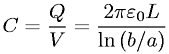Equations > Physics > Electricity and Magnetism > Capacitance of a cylindrical capacitor

### Capacitance of a cylindrical capacitorLatex Code:

MathML Code:

 $C=\fracQV=\frac2\pi {ϵ}_{0}L\mathrm{ln}\left(b\mathord/\vphantombaa\right)$

MathType 5.0: